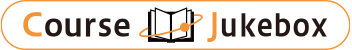### CourseDetailDegree
Bachelor
2
Course delivery methods
face-to-face
Subject
Biological sciences, Agriculture & related subjects, Physical sciences
Program
School
School of Life and Environmental Sciences
Department
Interdisciplinary Program in Life and Environmental Sciences
Campus
Tsukuba Campus
Classroom
2C403
Course Offering Year
2023-2024
Course Offering Month
April - June
Weekday and Period
Thu6
Capacity
Credits
1.0
Language
English
Course Number
EG02041

### Advanced Mathematics University of Tsukuba

#### Course Overview

In this course, students will have a short review of applied calculus and introduces with the advanced mathematics sections like geometrical meaning of differential equations, solution of ordinary and partial differential equations, numerical analysis and Laplace transformation. These advanced mathematical skills will be invaluable to them to interpret the concepts of modeling of real world problems related to life and environmental sciences.

#### Learning Achievement

After the successful completion of the course, students will be able to understand the expansion of functions, matrices and analyze differential equations numerically. They would be able to prepare a project using the matheical tools using matrix, differential equations and partial differential solutions of equations for creating model for life sciences.

#### Competence

Students entering in Advanced Mathematics should have a firm grasp of Calculus, linear algebra and vector calculus. They should be able to graph functions, integration and differentiation of functions, partial derivative of functions, optimization and evaluation of multiple integral.
In this course, students will learn the basics calculas and solution of differential equations. They will study standard functions withgraph, geometrical meaning of differential equations, modeling and solution of ordinary and partial differential equations. More generally, the students will improve their ability to think critically, to analyze a real problem and solve it using a wide array of mathematical tools. These skills will be invaluable to them in whatever path they choose to follow for study in the advance scienes and applications. They will also able to apply these ideas to a wide range of problems that include the life and environmental sciences issues. The students should be able to interpret the concepts of modeling algebraically, graphically, and verbally.

#### Course prerequisites

Mathematics background

Exam 50% (short answer questions), Quizzes and brief reports 30% and class participation including attitude 20%

#### Course schedule

Guidelines and details class outline will be given at the beginning of the class. The class is designed with hands on exercises and following sections:
Differentiation and Application of Differentiation:
Limits and Continuity, Average Rate of Change, Differentiation using Limits of Difference Quotients, Differentiation techniques; brief review. Instantaneous Rates of Change. Using first and second deriv
Exponential and Logarithmic Functions:
Exponential and logarithmic functions, Applications: Uninhibited Growth Model, Exponential Regression: Modeling world population growth, Applications of Decay. Extended life Science Examples- Maximum Sustainable Harv
Integration and Application of Integration:
Integration, Areas and Accumulations, A brief review of integration techniques, Extended examples from Numerical Analysis: Simpsons Rule, Trapezoidal Rule, and Calculating Volume. Extended life Science Example-
Matrices
Matrix operations: Matrix addition and subtraction, Matrix Algebra. Estimating Bird Population. Solving systems of linear equations, geometry of the linear systems: substitution method and elimination method. Inverse Matrix and Linear systems, De
Partial Derivatives and Applications:
Functions of several variables- find a function values for several variables- Body mass index, growth estimation. Partial derivatives-definitions, Approximate functions using partial derivatives examples of body mass
Ordinary Differential Equations and Applications:
Differential equations and Initial Value problems, Linear first order differential equations, Autonomous differential equations and stability, Logistic growth model, Logistic growth model with a threshold,
Numerical Solution of Deferential Equations- Numerical Analysis- Euler’s Method, Runge-Kutta Methods. Introductory discussion of Higher order Homogenous differential Equations. Project Exercise and Discussion. A project will be covered from a numerical/ma
Final Exam and Project Submission/Presentation
Project and Mathematical Modeling

Lectures

Tofael Ahamed

#### Other information

As discussed at Week 1.

#### Site for Inquiry

Link to the syllabus provided by the university